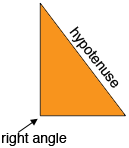SEARCH HOMEMath Central Quandaries & QueriesQuestion from brenae, a student: The Pythagorean Theorem, what is it ?Hi Brenae,

To state the Pythagorean Theorem in the usual way I need first to define a term. In a right triangle the longest side (the side opposite to the right angle) is called the hypotenuse.The classical statement of the Pythagorean Theorem is that:

In a right triangle the square of the hypotenuse is the sum of the squares of the other two sides.

In more modern language the statement is that:

In a right triangle the area of the square on the hypotenuse is the sum of the squares of the areas of the squares on the other two sides.Algebraically you could state the theorem.

If a right triangle has sides of length $a, b$ and $c$ units with the hypotenuse of length $c$ units then $a^2 + b^2 = c^2 .$

The converse of the Pythagorean Theorem is true also.

If a triangle has sides of length $a, b$ and $c$ units and $a^2 + b^2 = c^2$ then the triangle is a right triangle with the right angle where the sides of length $a$ and $b$ meet.

PennyMath Central is supported by the University of Regina and The Pacific Institute for the Mathematical Sciences.